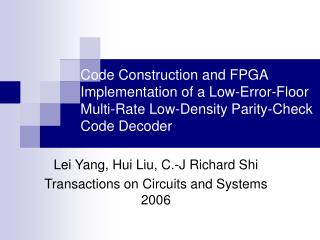DownloadDownload PresentationLei Yang, Hui Liu, C.-J Richard Shi Transactions on Circuits and Systems 2006

# Lei Yang, Hui Liu, C.-J Richard Shi Transactions on Circuits and Systems 2006

Download Presentation## Lei Yang, Hui Liu, C.-J Richard Shi Transactions on Circuits and Systems 2006

- - - - - - - - - - - - - - - - - - - - - - - - - - - E N D - - - - - - - - - - - - - - - - - - - - - - - - - - -
##### Presentation Transcript

1. Code Construction and FPGA Implementation of a Low-Error-Floor Multi-Rate Low-Density Parity-Check Code Decoder Lei Yang, Hui Liu, C.-J Richard Shi Transactions on Circuits and Systems 2006

2. Outline • Code Design and Rates • Log-BP and MSC • FUs • Result • Conclusion • Comment

3. Code Design and Rates • Regular rate 5/8 code • N=149 x 82 = 9536 • M=3 x 8 x 149 = 3576 • Regular rate 7/8 code • N=17 x 242 = 9792 • M=3 x 24 x 17 = 1224 • Irregular rate 1/2 code • N=251 x 36 = 9036 • M=18 x 251 = 4518

4. Code • Regular code • H3 consists of randomly located permutation matrix.

5. Irregular Code Nb x L = 36 x 251 Mb x L =18 x 251

6. Log-BP • Check Node Computation • Variable Node Computation

7. Min-Sum with Correction • Check Node Computation • Variable Node Computation

8. Finite Precision (6:3)

9. CNU (4CU)

10. VNU

11. Architecture

12. Nb x L = 36 x 251 Mb x L

13. Result • 40Mbps @ 100MHz (24 iterations) • 15Mbps @ 100MHz (60 iterations)

14. Conclusion • Offer a configurable 9-kbit multi-rate LDPC decoder. (00, 01 and 10 can work at rate 1/2, 5/8 and 7/8 respectively) • Archive BER 10-5 @ 1.4dB when irregular 1/2 is operating.

15. Comment • Min-Sum with Correction vs. Scaling Min-Sum • Irregular Code Decoding • Rate compatible Decoder

16. Comparison